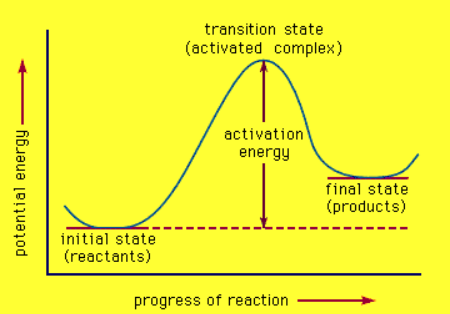# Factors affecting the rate of a reaction – Temperature

Chemical Kinetics: Factors affecting the rate of a reaction

Temperature

Rate of a reaction depends on the fraction of “effective collisions” per unit time. Effective collisions are those with proper orientation and enough energy to overcome the activation energy Ea barrier. When the reactant particles collide with the required activation energy, they form an activated complex. These unstable intermediate breaks down to form the products of the reaction. Thus, the rate depends on the activation energy and temperature. Higher activation energy means a high barrier where fewer reactant molecules form the transition-state complex. This leads to a slower rate of reaction. Higher temperature results in a larger fraction of reactant molecules with enough energy to overcome the energy barrier. This leads to a faster rate of reaction.

Temperature can be defined as a measure of the average kinetic energy of molecules in a substance. At any given temperature, a wide distribution of kinetic energies is observed. As the temperature increases, the Maxwell-Boltzmann curve flattens and broadens. Thus, at higher temperatures, a greater number of molecules have higher energy. If the dotted line in the graph below represents the activation energy, then as the temperature increases, the fraction of molecules that can overcome the activation energy barrier also increase. Usually, reactions speed up when the temperature increases (more energy into the system; therefore, more KE to overcome Ea have more effective collisions). Temperature causes molecules to move faster and cause collisions to occur more often, increasing the probability of effective collisions happening more often. Temp has no effect on lowering the activation energy Ea. It just gives more molecules the energy needed to overcome it thereby speeding up the reaction.Fig1: The Maxwell-Boltzmann Distribution

At any given temperature, the particles of a substance will have a range of kinetic energies and this can be shown on an energy distribution graph above. It shows that at the higher temperature (T2), many more molecules have energies greater than the activation energy and will be able to react when they collide. The fraction of molecules, f can be found through the Maxwell-Boltzmann expression:where R is the universal gas constant and T is the temperature in Kelvin.

A good “rule of thumb” is that reaction rate approximately doubles with a 10 °C rise in temperature. This is why storing food in a refrigerator slows down the reaction rate of spoiling food since a decrease in energy makes less effective collisions.Fig 2: Relationship between reaction rate and temperature

As shown in the figure above, increasing the temperature of the reactants increases the reaction rate because raising the kinetic energy of the reacting particles raises both the collision frequency and the collision energy. When the temperature of a reaction is increased, more of the collisions at high temperatures have sufficient energy to overcome the activation energy barrier and react in a faster manner.Fig 3: Energy profile of an endothermic reactionFig 4: Energy profile of an exothermic reaction

Likewise, whether a reaction is exothermic or endothermic, activation energy is required to initiate the reaction which is essentially obtained by increasing the temperature of the reactor to an optimum point where the particles are ready to collide and initiate the reaction. In general, terms, an increase in temperature increases the rate of reaction.